# 安卓自定义View进阶-Path之基本操作

2022-12-08 21:53:19 michael007js 56

# 二.Path详解

## Path含义

The Path class encapsulates compound (multiple contour) geometric paths consisting of straight line segments, quadratic curves, and cubic curves. It can be drawn with canvas.drawPath(path, paint), either filled or stroked (based on the paint’s Style), or it can be used for clipping or to draw text on a path.

Path封装了由直线和曲线(二次，三次贝塞尔曲线)构成的几何路径。你能用Canvas中的drawPath来把这条路径画出来(同样支持Paint的不同绘制模式)，也可以用于剪裁画布和根据路径绘制文字。我们有时会用Path来描述一个图像的轮廓，所以也会称为轮廓线(轮廓线仅是Path的一种使用方法，两者并不等价)

## Path使用方法详解### 第1组: moveTo、 setLastPoint、 lineTo 和 close

`Paint mPaint = new Paint();             // 创建画笔mPaint.setColor(Color.BLACK);           // 画笔颜色 - 黑色mPaint.setStyle(Paint.Style.STROKE);  // 填充模式 - 描边mPaint.setStrokeWidth(10);            // 边框宽度 - 10`

#### lineTo：

`public void lineTo (float x, float y)`

lineTo很简单，只有一个方法，作用也很容易理解，line嘛，顾名思义就是一条线。

`canvas.translate(mWidth / 2, mHeight / 2);// 移动坐标系到屏幕中心(宽高数据在onSizeChanged中获取)Path path = new Path();                     // 创建Pathpath.lineTo(200, 200);                    // lineTopath.lineTo(200,0);canvas.drawPath(path, mPaint);            // 绘制Path`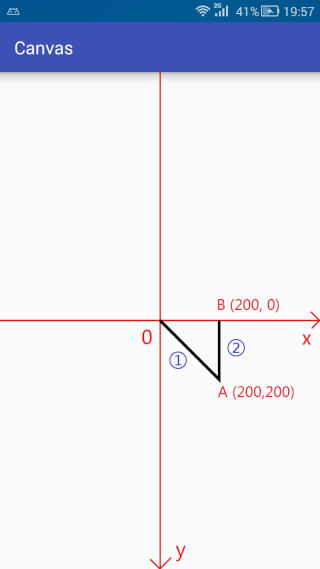#### moveTo 和 setLastPoint：

`// moveTopublic void moveTo (float x, float y)// setLastPointpublic void setLastPoint (float dx, float dy)`

`canvas.translate(mWidth / 2, mHeight / 2);// 移动坐标系到屏幕中心Path path = new Path();                     // 创建Pathpath.lineTo(200, 200);                    // lineTopath.moveTo(200,100);                       // moveTopath.lineTo(200,0);                         // lineTocanvas.drawPath(path, mPaint);            // 绘制Path`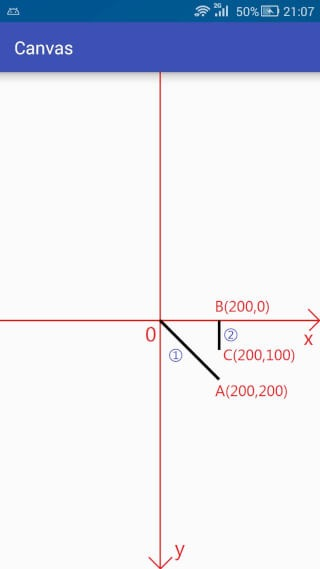moveTo只改变下次操作的起点，在执行完第一次LineTo的时候，本来的默认点位置是A(200,200),但是moveTo将其改变成为了C(200,100),所以在第二次调用lineTo的时候就是连接C(200,100) 到 B(200,0) 之间的直线(用蓝色圈2标注)。

`canvas.translate(mWidth / 2, mHeight / 2);// 移动坐标系到屏幕中心Path path = new Path();                     // 创建Pathpath.lineTo(200, 200);                    // lineTopath.setLastPoint(200,100);                 // setLastPointpath.lineTo(200,0);                         // lineTocanvas.drawPath(path, mPaint);            // 绘制Path`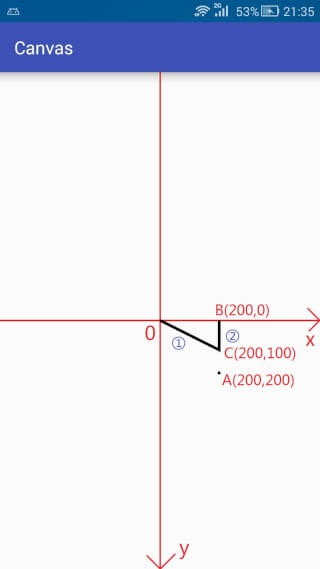setLastPoint是重置上一次操作的最后一个点，在执行完第一次的lineTo的时候，最后一个点是A(200,200),而setLastPoint更改最后一个点为C(200,100),所以在实际执行的时候，第一次的lineTo就不是从原点O到A(200,200)的连线了，而变成了从原点O到C(200,100)之间的连线了。

#### close

`public void close ()`

close方法用于连接当前最后一个点和最初的一个点(如果两个点不重合的话)，最终形成一个封闭的图形。

`canvas.translate(mWidth / 2, mHeight / 2);// 移动坐标系到屏幕中心Path path = new Path();                     // 创建Pathpath.lineTo(200, 200);                    // lineTopath.lineTo(200,0);                         // lineTopath.close();                               // closecanvas.drawPath(path, mPaint);            // 绘制Path`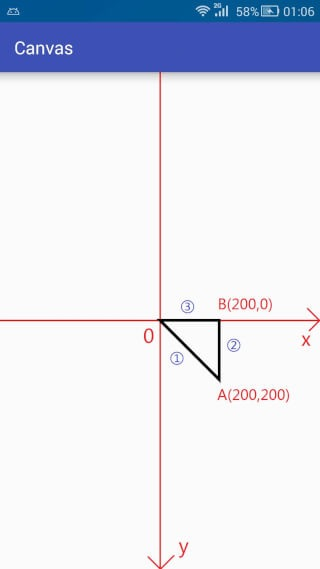#### 第一类(基本形状)

`// 第一类(基本形状)// 圆形public void addCircle (float x, float y, float radius, Path.Direction dir)// 椭圆public void addOval (RectF oval, Path.Direction dir)// 矩形public void addRect (float left, float top, float right, float bottom, Path.Direction dir)public void addRect (RectF rect, Path.Direction dir)// 圆角矩形public void addRoundRect (RectF rect, float[] radii, Path.Direction dir)public void addRoundRect (RectF rect, float rx, float ry, Path.Direction dir)`

Direction的意思是 方向，趋势。 点进去看一下会发现Direction是一个枚举(Enum)类型，里面只有两个枚举常量，如下：

`canvas.translate(mWidth / 2, mHeight / 2);// 移动坐标系到屏幕中心Path path = new Path();path.addRect(-200,-200,200,200, Path.Direction.CW);canvas.drawPath(path,mPaint);`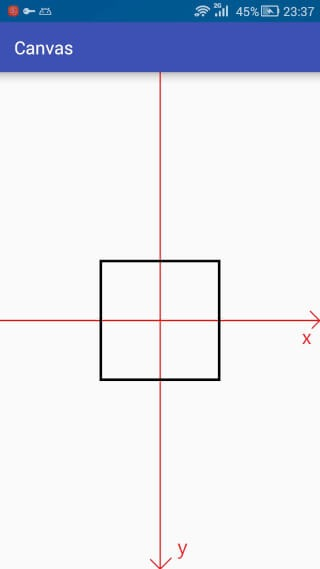(╯°Д°)╯︵ ┻━┻(再TM掀一次) 坑人也不带这样的啊，一毛一样要它干嘛。

`canvas.translate(mWidth / 2, mHeight / 2);// 移动坐标系到屏幕中心Path path = new Path();path.addRect(-200,-200,200,200, Path.Direction.CW);path.setLastPoint(-300,300);              // <-- 重置最后一个点的位置canvas.drawPath(path,mPaint);`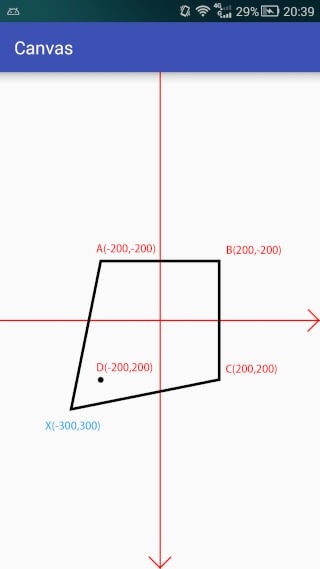`canvas.translate(mWidth / 2, mHeight / 2);// 移动坐标系到屏幕中心Path path = new Path();path.addRect(-200,-200,200,200, Path.Direction.CCW);path.setLastPoint(-300,300);              // <-- 重置最后一个点的位置canvas.drawPath(path,mPaint);`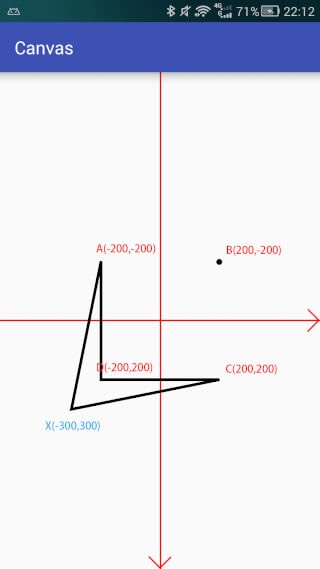#### 第二类(Path)

`// 第二类(Path)// pathpublic void addPath (Path src)public void addPath (Path src, float dx, float dy)public void addPath (Path src, Matrix matrix)`

`canvas.translate(mWidth / 2, mHeight / 2);// 移动坐标系到屏幕中心canvas.scale(1,-1);                         // <-- 注意 翻转y坐标轴Path path = new Path();Path src = new Path();path.addRect(-200,-200,200,200, Path.Direction.CW);src.addCircle(0,0,100, Path.Direction.CW);path.addPath(src,0,200);mPaint.setColor(Color.BLACK);           // 绘制合并后的路径canvas.drawPath(path,mPaint);`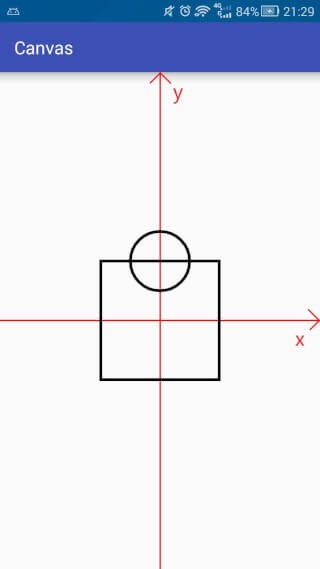`// 第三类(addArc与arcTo)// addArcpublic void addArc (RectF oval, float startAngle, float sweepAngle)// arcTopublic void arcTo (RectF oval, float startAngle, float sweepAngle)public void arcTo (RectF oval, float startAngle, float sweepAngle, boolean forceMoveTo)`

PS: sweepAngle取值范围是 [-360, 360)，不包括360，当 >= 360 或者 < -360 时将不会绘制任何内容， 对于360，你可以用一个接近的值替代，例如: 359.99。

forceMoveTo是什么作用呢？

`canvas.translate(mWidth / 2, mHeight / 2);// 移动坐标系到屏幕中心canvas.scale(1,-1);                         // <-- 注意 翻转y坐标轴Path path = new Path();path.lineTo(100,100);RectF oval = new RectF(0,0,300,300);path.addArc(oval,0,270);// path.arcTo(oval,0,270,true);             // <-- 和上面一句作用等价canvas.drawPath(path,mPaint);`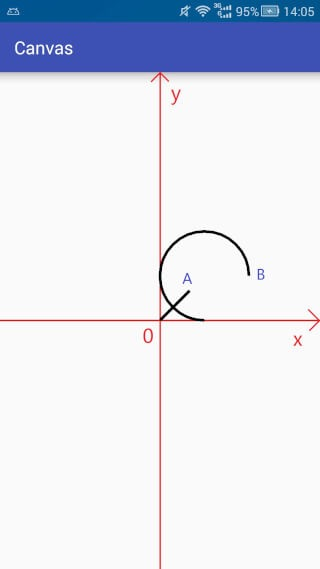`canvas.translate(mWidth / 2, mHeight / 2);// 移动坐标系到屏幕中心canvas.scale(1,-1);                         // <-- 注意 翻转y坐标轴Path path = new Path();path.lineTo(100,100);RectF oval = new RectF(0,0,300,300);path.arcTo(oval,0,270);// path.arcTo(oval,0,270,false);             // <-- 和上面一句作用等价canvas.drawPath(path,mPaint);`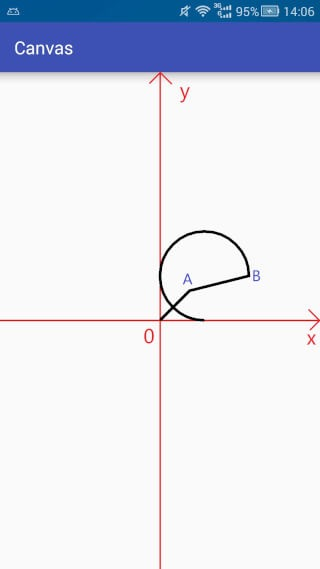### 第3组：isEmpty、 isRect、isConvex、 set 和 offset

#### isEmpty

`public boolean isEmpty ()`

`Path path = new Path();Log.e("1",path.isEmpty()+"");path.lineTo(100,100);Log.e("2",path.isEmpty()+"");`

log输出结果:

`com.sloop.canvas E/1: truecom.sloop.canvas E/2: false`

#### isRect

`public boolean isRect (RectF rect)`

`path.lineTo(0,400);path.lineTo(400,400);path.lineTo(400,0);path.lineTo(0,0);RectF rect = new RectF();boolean b = path.isRect(rect);Log.e("Rect","isRect:"+b+"| left:"+rect.left+"| top:"+rect.top+"| right:"+rect.right+"| bottom:"+rect.bottom);`

log 输出结果:

`com.sloop.canvas E/Rect: isRect:true| left:0.0| top:0.0| right:400.0| bottom:400.0`

#### set

`public void set (Path src)`

`canvas.translate(mWidth / 2, mHeight / 2);// 移动坐标系到屏幕中心canvas.scale(1,-1);                         // <-- 注意 翻转y坐标轴Path path = new Path();                     // path添加一个矩形path.addRect(-200,-200,200,200, Path.Direction.CW);Path src = new Path();                    // src添加一个圆src.addCircle(0,0,100, Path.Direction.CW);path.set(src);                            // 大致相当于 path = src;canvas.drawPath(path,mPaint);`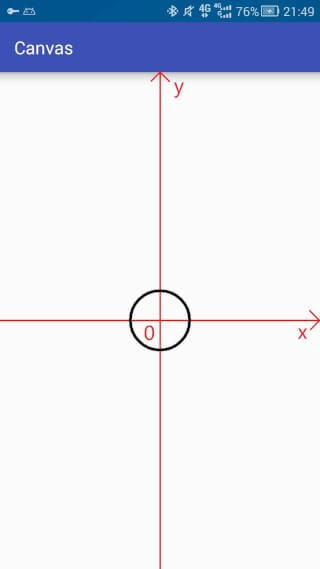#### offset

`public void offset (float dx, float dy)public void offset (float dx, float dy, Path dst)`

`canvas.translate(mWidth / 2, mHeight / 2);// 移动坐标系到屏幕中心canvas.scale(1,-1);                         // <-- 注意 翻转y坐标轴Path path = new Path();                     // path中添加一个圆形(圆心在坐标原点)path.addCircle(0,0,100, Path.Direction.CW);Path dst = new Path();                    // dst中添加一个矩形dst.addRect(-200,-200,200,200, Path.Direction.CW);path.offset(300,0,dst);                     // 平移canvas.drawPath(path,mPaint);               // 绘制pathmPaint.setColor(Color.BLUE);              // 更改画笔颜色canvas.drawPath(dst,mPaint);              // 绘制dst`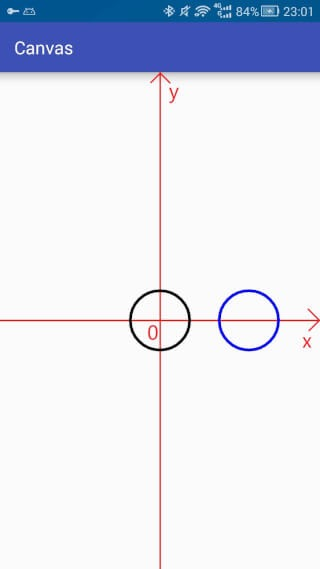# 三.总结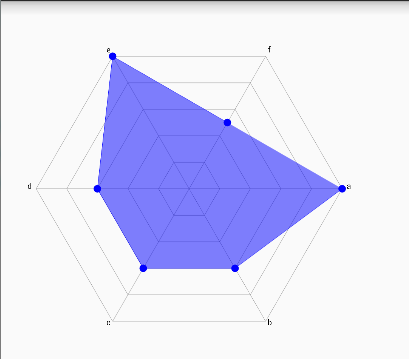(,,• ₃ •,,)

PS: 由于本人水平有限，某些地方可能存在误解或不准确，如果你对此有疑问可以提交Issues进行反馈。